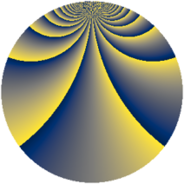# Properties

 Label 1520.2.cyLevel $1520$ Weight $2$ Character orbit 1520.cy Rep. character $\chi_{1520}(349,\cdot)$ Character field $\Q(\zeta_{12})$ Dimension $944$ Sturm bound $480$

# Related objects

## Defining parameters

 Level: $$N$$ $$=$$ $$1520 = 2^{4} \cdot 5 \cdot 19$$ Weight: $$k$$ $$=$$ $$2$$ Character orbit: $$[\chi]$$ $$=$$ 1520.cy (of order $$12$$ and degree $$4$$) Character conductor: $$\operatorname{cond}(\chi)$$ $$=$$ $$1520$$ Character field: $$\Q(\zeta_{12})$$ Sturm bound: $$480$$

## Dimensions

The following table gives the dimensions of various subspaces of $$M_{2}(1520, [\chi])$$.

Total New Old
Modular forms 976 976 0
Cusp forms 944 944 0
Eisenstein series 32 32 0

## Trace form

 $$944q - 4q^{4} - 2q^{5} - 4q^{6} + O(q^{10})$$ $$944q - 4q^{4} - 2q^{5} - 4q^{6} - 8q^{10} - 16q^{11} - 12q^{14} - 4q^{15} + 20q^{20} - 16q^{21} + 4q^{24} - 24q^{26} - 4q^{29} - 72q^{30} + 64q^{31} - 12q^{34} + 12q^{35} - 20q^{36} - 12q^{40} - 60q^{44} - 40q^{45} - 32q^{46} + 816q^{49} + 4q^{50} - 36q^{51} - 36q^{54} - 128q^{56} - 4q^{59} - 20q^{60} + 28q^{61} - 40q^{64} - 16q^{65} + 44q^{66} + 72q^{69} - 52q^{70} - 4q^{74} + 116q^{75} + 84q^{76} - 120q^{79} - 34q^{80} + 400q^{81} - 128q^{84} - 22q^{85} + 28q^{86} - 14q^{90} - 32q^{91} - 200q^{94} - 32q^{95} - 120q^{96} - 24q^{99} + O(q^{100})$$

## Decomposition of $$S_{2}^{\mathrm{new}}(1520, [\chi])$$ into newform subspaces

The newforms in this space have not yet been added to the LMFDB.